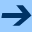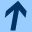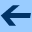On the formal first cocycle equation for iteration groups of type II

## On the formal first cocycle equation for iteration groups of type II

Jointly written with LUDWIG REICH.

ESAIM: Proceedings 36, 32-47, 2012. Proceedings of the European Conference on Iteration Theory 2010.

Abstract: Let x be an indeterminate over ℂ. We investigate solutions

 α(s,x)=∑n≥ 0 αn(s)xn,..
αn: ℂ→ ℂ, n≥ 0, of the first cocycle equation
(Co1) α(s+t,x)= α(s,x)α(t,F (s,x)),        s,t∈ ℂ,
in C[[x]], the ring of formal power series over ℂ, where (F(s,x))s∈ ℂ is an iteration group of type II, i.e. it is a solution of the translation equation
(T) F(s+t,x)=F(s,F(t,x)),        s,t∈ ℂ,
of the form F(s,x)≡ x+ck(s)xkmodxk+1, where k≥ 2 and ck ≠ 0 is necessarily an additive function. It is easy to prove that the coefficient functions αn(s) of
 α(s,x)=1+∑n≥ 1αn(s)xn..
are polynomials in ck(s).

It is possible to replace this additive function ck by an indeterminate. Finally, we obtain a formal version of the first cocycle equation in the ring (ℂ[y])[[x]]. We solve this equation in a completely algebraic way, by deriving formal differential equations or an Aczél-Jabotinsky type equation. This way it is possible to get the structure of the coefficients in great detail which are now polynomials. We prove the universal character of these polynomials depending on certain parameters, the coefficients of the generator K of a formal cocycle for iteration groups of type II. Rewriting the solutions Γ(y,x) of the formal first cocycle equation in the form n≥ 1ψn(x)yn as elements of (ℂ[[x]])[[y]], we obtain explicit formulas for ψn in terms of the derivatives H(j)(x) and K(j)(x) of the generators H and K and also a representation of Γ(y,x) similar to a Lie-Gröbner series. There are interesting similarities between the solutions G(y,x) of the formal translation equation for iteration groups of type II and the solutions Γ(y,x) of the formal first cocycle equation for iteration groups of type II.

harald.fripertinger "at" uni-graz.at, March 4, 2019